### 所属分类：学习宝典　发布时间：2018-04-30　阅读次数：2975 次

1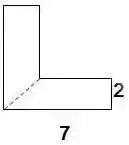S组=（7-2+7）×2÷2×2=24（平方厘米）

▌例2：下列两个正方形边长分别为8厘米和5厘米，求阴影部分面积。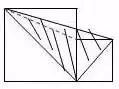S=5×5÷2+5×8÷2+（8-5）×5÷2=12.5+20+7.5=38（平方厘米）

▌例3：左图中两个正方形边长分别为8厘米和6厘米。求阴影部分面积。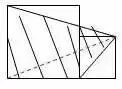S阴=8×（8+6）÷2+8×6÷2=56+24=80（平方厘米）

2

▌例1：已知正方形边长4厘米，A、B、C、D是正方形边上的中点，P是任意一点。求阴影部分面积。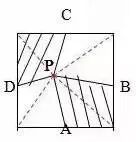S阴=4×4÷2=8（平方厘米）

▌例2：将下图平行四边形分成三角形和梯形两部分，它们面积相差40平方厘米，平行四边形底20.4厘米，高8厘米。梯形下底是多少厘米？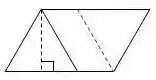▌例3：平行四边形的面积是48平方厘米，BC分别是这个平行四边形相邻两条边的中点，连接A、B、C得到4个三角形。求阴影部分的面积。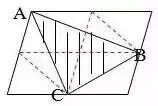S阴=48÷8×3=18（平方厘米）

3

▌例1：已知OC=2AO,SABO=2㎡，求梯形ABCD的面积。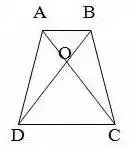SDOC=4×2=8（㎡）
SABCD=2+4×2+8=18（㎡）

▌例2：已知S阴=8.75㎡，求下图梯形的面积。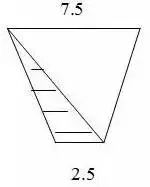S=8.75×（3+1）=35（㎡）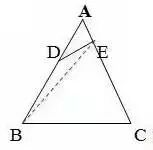SABE=1×3=3  SABC=3×5=15

4

▌例1：已知S阴=20㎡，EF为中位线求梯形ABCD的面积。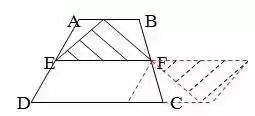▌例2：求下图面积（单位厘米）。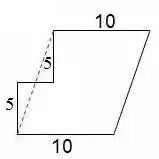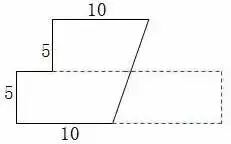▌例3：把一个长方形的长和宽分别增加2厘米，面积增加24平方厘米。求原长方形的周长。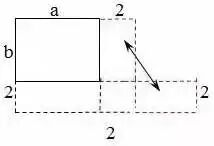5

▌例1：已知AB平行于EC，求阴影部分面积。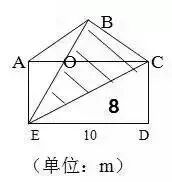▌例2：下图两个正方形边长分别是6分米、4分米。求阴影部分面积。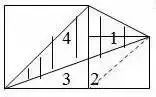6

▌例1：已知长方形周长为22厘米，长7厘米，求阴影部分面积。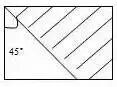S阴=（7+（7-4））×4÷2=20（平方厘米）

▌例2：已知下列两个等腰直角三角形，直角边分别是10厘米和6厘米。求阴影部分的面积。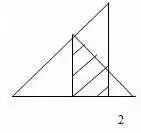S阴=（6+2）×4÷2=16（厘米）

▌例3：下图长方形长9厘米，宽6厘米，求阴影部分面积。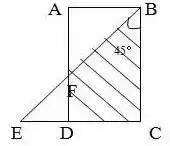FD=ED=9-6=3（厘米）
S阴=（9+3）×6÷2=36（平方厘米）

7

▌例：求左下图的面积（单位：米）。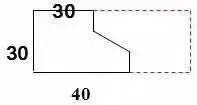S=（40+30）×30÷2=1050（平方米）

8

▌例1：图中三角形甲的面积比乙的面积少8平方厘米，AB=8cm，CE=6cm。求三角形甲和三角形乙的面积各是多少？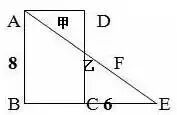8X+8=8（6+X）÷2
X=4
4Y÷2+8=6（8-Y）÷2
Y=3.2
S甲=4×3.2÷2=6.4（c㎡）
S乙=6.4+8=14.4（c㎡）

▌例2：下图是一个等腰三角形，它的腰长是20厘米，面积是144平方厘米。在底边上任取一点向两腰作垂线，得a和b，求a+b的和。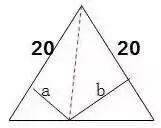20b÷2+20a÷2=144
10a+10b=144

a+b=14.4

9

▌例1：下图两个正方形的边长分别是6厘米和3厘米，求阴影部分的面积。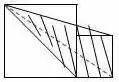S阴=S△+S△
=3×（6+3）÷2+3×6÷2
=22.5（平方厘米）

▌例2：下图长方形长10厘米，宽7厘米，求阴影部分面积。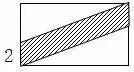S阴=10×2=20（平方厘米）
• 电话咨询

400-8821-211
• 关注我们